# Card models of polyhedra

For many years I have constructed card models (and a few stick models) of polyhedra. A selection of these are shown below and images of more may be added in future. For each model shown an indication is given of the scale of the original model, as this is not generally apparent from photographs. Where I have made multiple models of one polyhedron, only one is generally shown here. Not all models I have made are in my current collection.

Other sites of polyhedron makers include that of Magnus Wenninger. Books on the subject include Polyhedron Models and Dual Models by Wenninger, Mathematical Models by Cundy and Rollett and The Fifty-Nine Icosahedra by Coxeter, Du Val, Flather and Petrie.

## Platonic solids

Tetrahedron (edge length 5cm):Cube or hexahedron (edge length 5cm):Octahedron (edge length 5cm):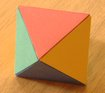Dodecahedron (edge length 5cm):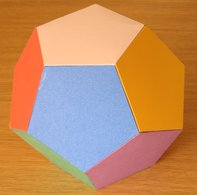Icosahedron (edge length 5cm):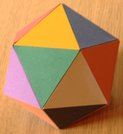## Archimedean polyhedra

Truncated tetrahedron (edge length 5cm):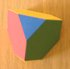Truncated cube or truncated hexahedron (edge length 3cm):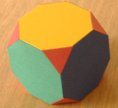Truncated octahedron (edge length 5cm):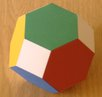Truncated dodecahedron (edge length 3cm):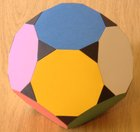Truncated icosahedron (edge length 3cm):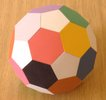Cuboctahedron (edge length 5cm):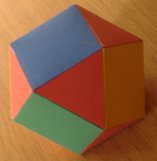Icosidodecahedron (edge length 5cm):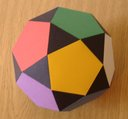Rhombicuboctahedron or small rhombicuboctahedron (edge length 5cm):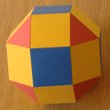Rhombicosidodecahedron or small rhombicosidodecahedron (edge length 3cm):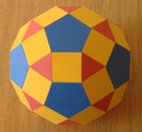Rhombitruncated cuboctahedron or great rhombicuboctahedron (edge length 3cm):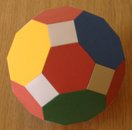Rhombitruncated icosidodecahedron or great rhombicosidodecahedron (edge length 3cm):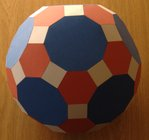Snub cube or snub hexahedron (edge length 5cm):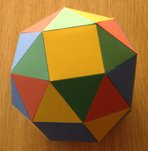Snub dodecahedron (edge length 3cm):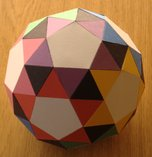## Kepler-Poinsot polyhedra

Small stellated dodecahedron (edge length 15cm):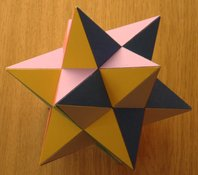Great dodecahedron (edge length 10cm):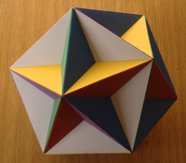Great stellated dodecahedron (edge length 25cm):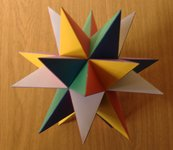Great icosahedron (edge length 25cm):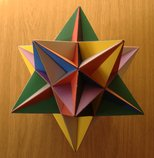## Other nonconvex uniform polyhedra

I have made models of a small subset of these. Images of some are shown here and others will appear here in future. There are no standard names for these polyhedra; the names given here are from Polyhedron Models, or where two names are given the first is from Polyhedron Models and the second is from Dual Models.

Tetrahemihexahedron (edge length 8cm):Octahemioctahedron (edge length 8cm):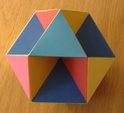Cubohemioctahedron (edge length 8cm):Small cubicuboctahedron (edge length 5cm):Small rhombihexahedron (edge length 5cm):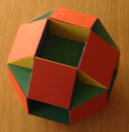Great cubicuboctahedron (edge length 15cm):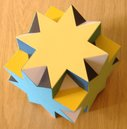Great rhombihexahedron (edge length 15cm):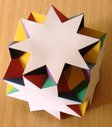Quasitruncated hexahedron or stellated truncated hexahedron (edge length 15cm):Cuboctatruncated cuboctahedron or cubitruncated cuboctahedron (edge length 8cm):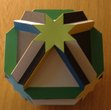Quasirhombicuboctahedron or great rhombicuboctahedron (edge length 15cm):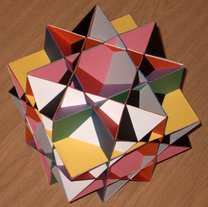Dodecadodecahedron (edge length 8cm):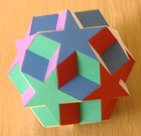Truncated great dodecahedron or great truncated dodecahedron (edge length 6cm):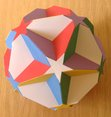Great icosidodecahedron (edge length 12cm):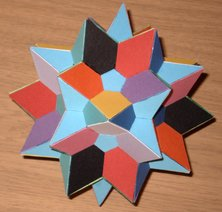Great icosihemidodecahedron (edge length 12cm):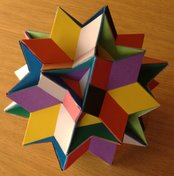Small ditrigonal icosidodecahedron (edge length 5cm):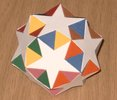Quasitruncated small stellated dodecahedron or small stellated truncated dodecahedron (edge length 8cm):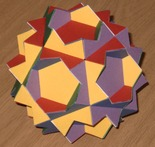Small icosicosidodecahedron (edge length 4cm):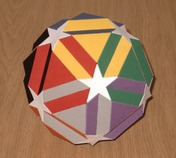Ditrigonal dodecahedron or ditrigonal dodecadodecahedron (edge length 5cm):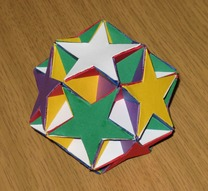Quasitruncated great stellated dodecahedron or great stellated truncated dodecahedron (edge length 8cm):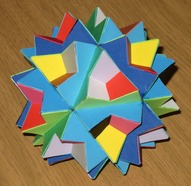Small dodecicosidodecahedron (edge length 2cm):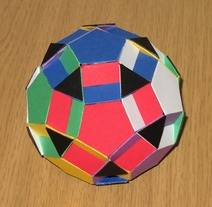## Other polyhedra

Rhombic dodecahedron (short face diagonal 3cm):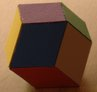First stellation of rhombic dodecahedron (short face diagonal 3cm):Second stellation of rhombic dodecahedron (short face diagonal 3cm):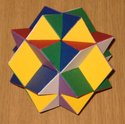Final stellation of rhombic dodecahedron (short face diagonal 3cm):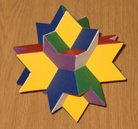Rhombic triacontahedron (short face diagonal 3cm):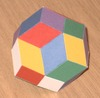Rhombic hexecontahedron (short face diagonal 3cm):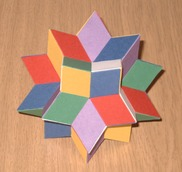Stella octangula (octahedron edge length 5cm):Compound of five cubes (cube edge length 12cm):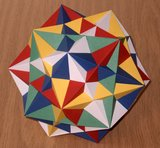Compound of five octahedra (octahedron edge length 8cm):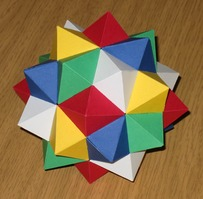Compound of five tetrahedra (tetrahedron edge length 12cm):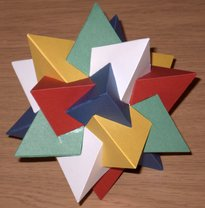First stellation of icosidodecahedron (icosidodecahedron edge length 2cm):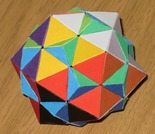First stellation of rhombicuboctahedron (rhombicuboctahedron edge length 2cm):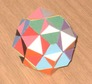Triakis tetrahedron (tetrahedron edge length 5cm):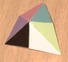Triakis octahedron (octahedron edge length 5cm):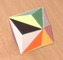Tetrakis cube (cube edge length 5cm):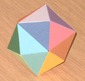Compound of three octahedra (octahedron edge length 6cm):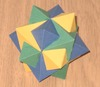Compound of three cubes (cube edge length 6cm):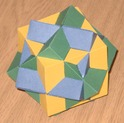Compound of four cubes (cube edge length 6cm):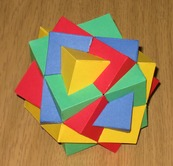Compound of four octahedra (octahedron edge length 10cm):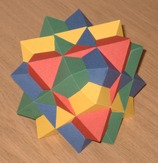I have made various other polyhedra, images of some of which may appear here in future.